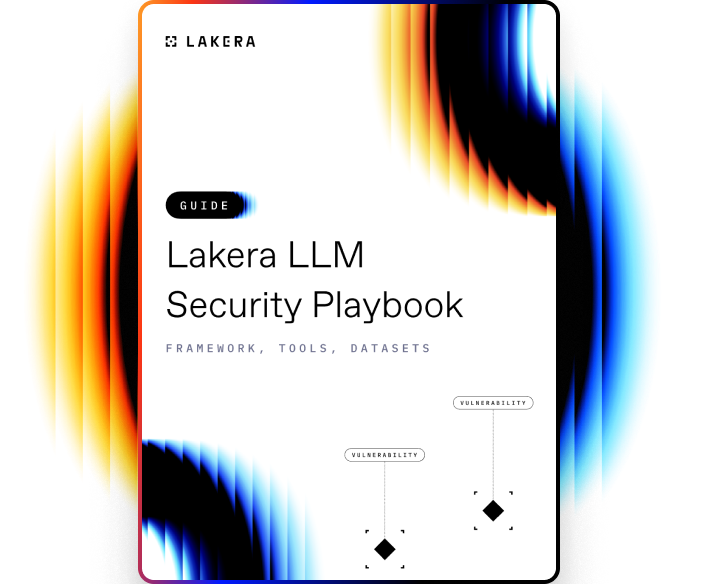VOCABULARY

# Cross-Entropy Loss

Cross-entropy loss is a cost function used in machine learning algorithms where the task is to classify objects into multiple classes. It is a measure of the difference between two probability distributions, often the true data distribution and the estimated distribution produced by a machine learning model. The goal is to minimize the entropy loss to improve the accuracy of the model's predictions.

## How Cross-Entropy Loss works

Cross-entropy loss is calculated using the probabilities output by the model (for each class in a multi-class classification problem) and the true label of the instance. If the predicted probabilities match the actual labels perfectly, the entropy loss is zero. Conversely, if the predictions are entirely incorrect, the loss tends towards infinity.

The formula for calculating cross-entropy loss for binary classification is given by:

-[y*log(p) + (1-y)*log(1-p)]

where y is the true label (0 or 1), and p is the predicted probability of the instance being in class 1.

By minimizing the entropy loss, the model's predicted probability distribution gets closer to the true distribution, improving the model's overall performance on the data.Learn how to protect against the most common LLM vulnerabilities

Download this guide to delve into the most common LLM security risks and ways to mitigate them.

Related termsActivate
untouchable mode.